## 1. Introduction

The Towers of Hanoi is a classic mathematical puzzle that has applications in both computer science and mathematics. Originally invented by a French mathematician named Édouard Lucas, this puzzle illustrates the power and elegance of recursion.

In this article, we’ll study algorithms and the complexity of the Towers of Hanoi problem. We’ll start by explaining what the problem is using a detailed example. Then we’ll move on to a discussion of associated algorithms and complexity.

We’ll present both a recursive and an iterative solution to the problem as well as a pseudocode for the recursive algorithm. Finally, we’ll give a detailed complexity analysis.

## 2. Problem Explanation

There are three rods anddisks of different sizes. Any disk can slide onto any rod. The initial state of the puzzle has all the disks on one rod in decreasing order of size from bottom to top, with the largest disk being at the bottom.

The goal of the puzzle is to move the entire initial stack to another rod while following three simple rules:

• A disk cannot be placed on top of another disk whose size is smaller
• Only one disk can be moved at a time
• In each move, we must take the uppermost disk from one of the stacks and move it on top of another stack or to an empty rod

Let’s see an example of the puzzle in action when there are a total of three disks. We denote the rods by A, B, and C. We start with all three disks stacked on one of the three rods (rod A in this case):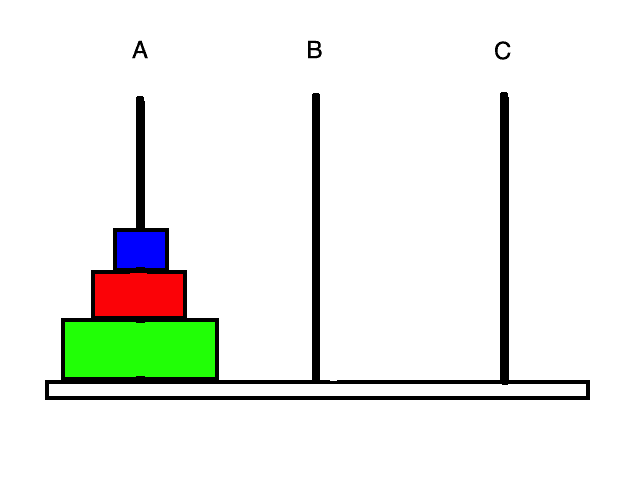Let’s say the goal is to move all three disks to rod C (which rod we choose as our destination doesn’t matter). Our first move can only involve the smallest disk since there is only one stack and this disk is at the top of it. So let’s move the smallest disk to rod C: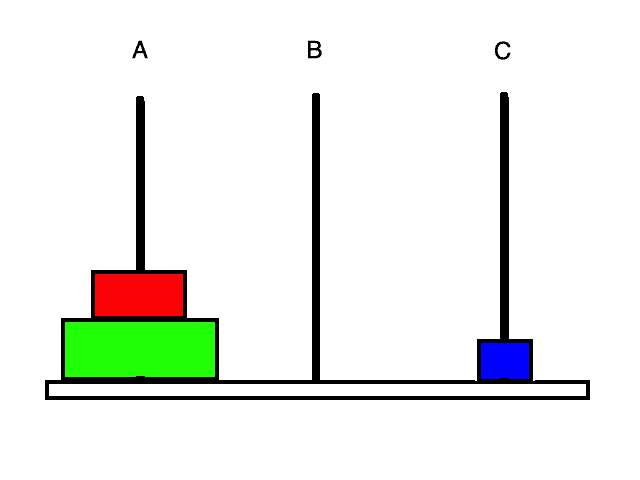Now we have two disks that we can move, namely the smallest and the second smallest. Moving the smallest disk will not accomplish much since moving it to rod A gets us back to the original state, and moving it to rod B is redundant since we could have just moved the smallest disk to rod B in the first place.

So, it’s clear that we should move the second smallest disk. Let’s move it to rod B: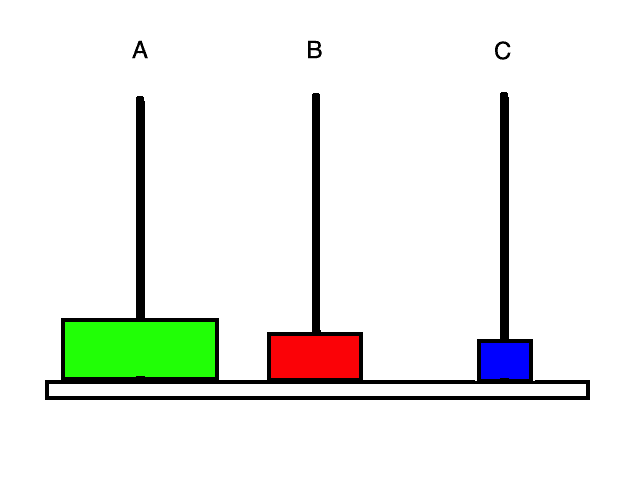Now let’s move the smallest disk to rod B as well: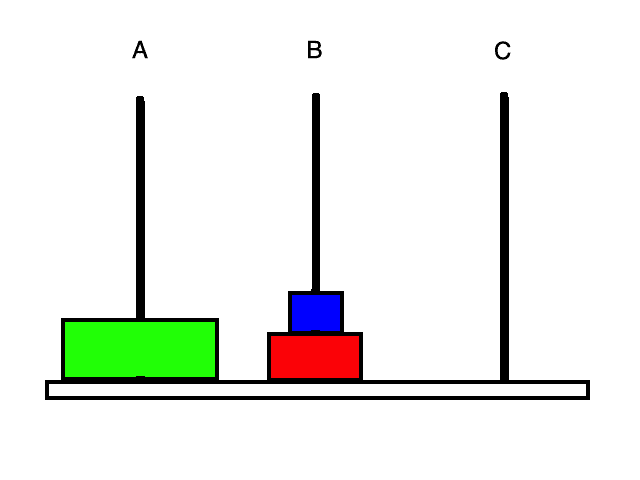We know that we want the largest disk at the bottom of rod C so our next move is to move it there: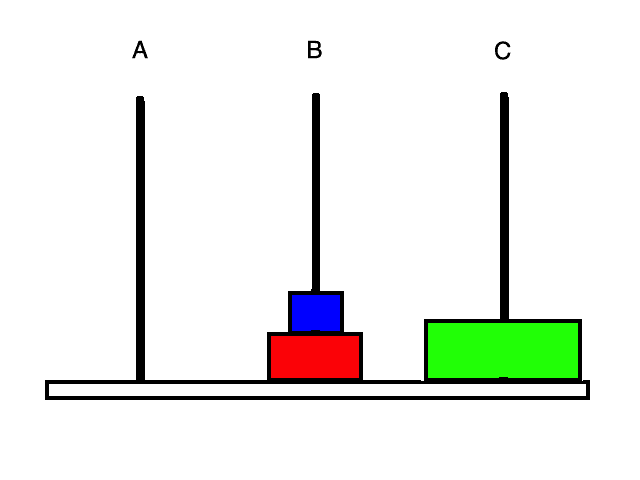Now we need to move the stack on rod B to rod C. To do this we can first move the smallest disk to rod A: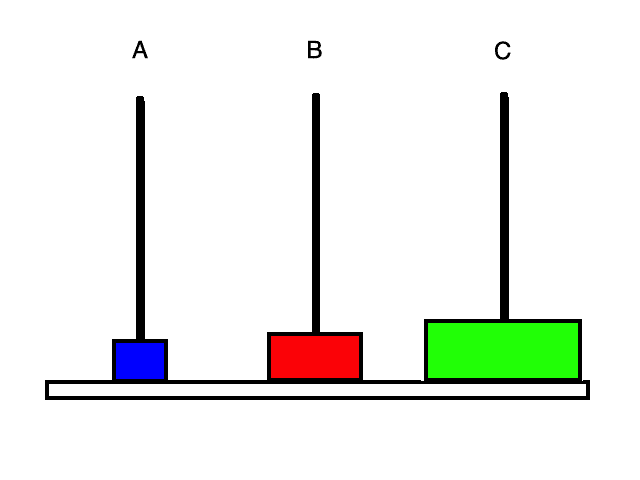Then we can move the second smallest disk to rod C: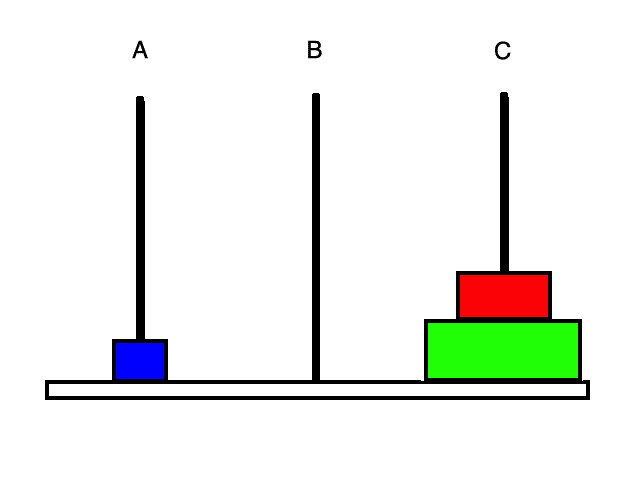Finally, we move the smallest disk to rod C as well and we are done!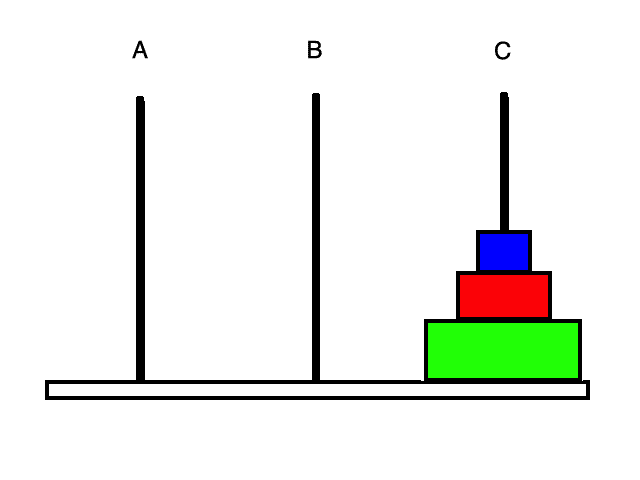If we take a closer look at what we just did, we’ll notice that the problem has a naturally recursive structure. First, we moved the top two disks to rod B, then we moved the largest disk to rod C, and then we once again moved the smallest two disks but this time to rod C. This pattern suggests a recursive solution that we can easily implement.

## 3. Algorithms

### 3.1. Recursive Algorithm

We can solve this problem recursively because we can break it down into a set of smaller subproblems.

When we want to move a stack of disks from a source rod A to a destination rod C, we can use this four-step process:

1. If there is only one disk, simply move it to rod C and exit
2. Recursively move the topdisks from rod A to rod B
3. Move the leftover largest disk on rod A to rod C
4. Recursively move the stack of disks from rod B to rod C

If we look at steps 1 and 3, it’s not too difficult to see that they are solving the same problem as our original problem, except that there aredisks to move instead of. It’s the same problem because we are moving a stack of disks from one rod to another rod, and any disk can be put on top of the largest disk.

### 3.2. Iterative Algorithm

Another way to solve this problem is to think about it iteratively.

The idea behind this method is to choose which pair of rods to make a legal move between, depending on what iteration of the algorithm we are on. This approach is not as intuitive as the recursive method but it works nevertheless.

Once again we have four inputs which are the same as in the recursive approach:

1. Compute the minimum number of moves needed to solve the puzzle, which is equal to(we will see later how we arrived at this number). Letbe equal to this value.
2. Ifis even then set the destination rod to the auxiliary rod and the auxiliary rod to the original destination rod. In other words, exchangeand.
3. Iterate in a loop fromto. In each iteration of this loop, we do something depending on what remainder we get when we divideby three. If i % 3 == 1, make the legal move between rodsand. If i % 3 == 2, make the legal move between rodsand. If i % 3 == 0, make the legal move between rodsand.

## 4. Pseudocode

We can now write a nice recursive algorithm for this problem using pseudocode. This algorithm will print out the solution to the given Towers of Hanoi instance by printing out step-by-step directions.

We have four inputs:,,, and.is the number of disks,is the name of the source rod,is the name of the auxiliary rod, andis the name of the destination rod:## 5. Complexity

We know that the time taken by an algorithm will be proportional to the number of elementary moves made. In our case, an elementary move is to move a disk from one rod to another rod. Therefore, the time taken by an algorithm for Towers of Hanoi will be proportional to the number of times we move some disk.

Letdenote the minimum number of disk moves needed to solve a Towers of Hanoi instance withdisks. Our goal in the complexity analysis of this problem is to obtain an expression forin terms of, without any recursion in the expression.

First, we notice thatequals one since if there is one disk we can simply move it to the destination rod in one move. Now if,is equal tobecause we can first recursively move the topdisks to the auxiliary rod, move the largest disk to the destination rod, and then recursively move the stack from the auxiliary rod to the destination rod.

How do we know thatis the minimum number of moves? We can prove this formally by mathematical induction: For the base case of= 1, we have seen thatequals one. This is the minimum number of moves because we cannot do better than one move for a single disk.

Then for the inductive case, we can first assume thatis minimum for. We know that we’ll have to at least move the topdisks out of the way with the minimum number of moves possible and then move the largest disk to the destination rod. By our induction hypothesis we know we can achieve this in a minimum ofmoves.

Then we still need to move the remaining disks to the destination rod but with the minimum possible number of moves. Therefore, our total moves ofmust be the minimum.

Now we know thatis the minimum but we still would like to get an expression forthat does not have any recursion in it. In other words, we would like to solve this recurrence relation.

Let’s first see if we can find a pattern in the values for. We notice that= 1,= 3,= 7,= 15,= 31, and so on. It seems likeequals something like. Let’s use a guess and check method to verify whether this guess is correct:so our hypothesis holds for the base case. Now assume that our hypothesis holds for all smaller values of. Then by using the inductive hypothesis we know that, so our guess was correct!.

There are other ways we can solve this recurrence such as using the Master Theorem or by unrolling the recurrence.

We have seen that the minimum number of moves required for a Towers of Hanoi instance withdisks is. This isand grows very fast asincreases.

To get a sense of how bad this time complexity is, suppose it takes us one second to move one disk from a rod to another rod. Then to solve an instance with 64 disks it will take us about 585 billion years!

## 6. Conclusion

In this article, we first introduced and explained the Towers of Hanoi problem.

Then we provided both a recursive and an iterative algorithm for solving it and showed pseudocode for the recursive solution.

Finally, we gave a complexity analysis. We saw that to solve this puzzle we need to perform at leaststeps.